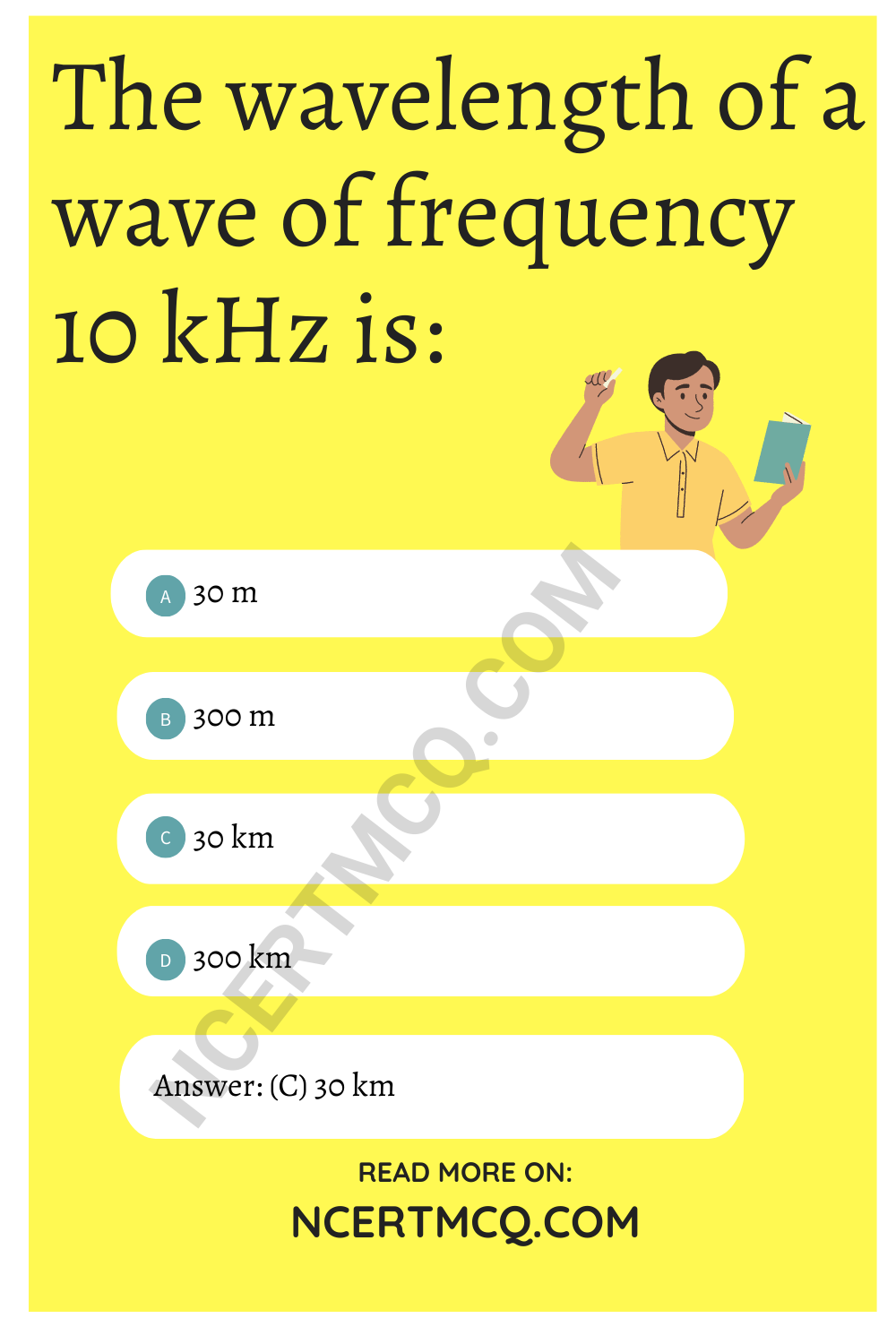Check the below NCERT MCQ Questions for Class 12 Physics Chapter 15 Communication Systems with Answers Pdf free download. MCQ Questions for Class 12 Physics with Answers were prepared based on the latest exam pattern. We have provided Communication Systems Class 12 Physics MCQs Questions with Answers to help students understand the concept very well.

## Communication Systems Class 12 MCQs Questions with Answers

MCQ On Communication System Class 12 Question 1.
Who undertook the first space walk and in which year?
(a) Leonov 1965
(b) Neil Armstrong, 1969
(c) Rakesh Sharma. 1998
(d) None of these

MCQ On Communication System Class 12 Pdf Question 2.
The term used “to collect the information about an object and a place without physical contact” is called :
(a) modulation
(b) communication
(c) amplification
(d) remote sensing

Communication System Class 12 MCQ Question 3.
The velocity of electromagnetic wave is:
(a) 3 × 105 ms-1
(b) 3 × 106 ms-1
(c) 3 × 108 ms-1
(d) 3 × 1010 ms-1

Answer: (c) 3 × 108 ms-1

Chapter 15 Physics Class 12 MCQs Question 4.
The audio frequencies range from:
(a) 20 Hz to 20.000 kHz
(b) 20 Hz to 20 kHz
(c) 20 kHz to 20.000 kHz
(d) None of these

Answer: (b) 20 Hz to 20 kHz

MCQ On Communication System Question 5.
The wavelength of a wave of frequency 10 kHz is:
(a) 30 m
(b) 300 m
(c) 30 km
(d) 300 kmCommunication Systems MCQ Question 6.
The area served by an antenna of height 100 m is:
(a) 109
(b) 4 × 109
(c) 6 × 109
(d) 8 × 1010

Communication System MCQ Pdf Question 7.
Which of the following is not transducer?
(a) Loudspeaker
(b) Amplifier
(c) Microphone
(d) All

MCQ On Communication Question 8.
The space waves which are affected seriously by atmospheric conditions are:
(a) MF
(b) HUF
(c) VHF
(d) UHF

Communication System MCQ Questions Question 9.
An antenna is:
(a) Inductive
(b) Capacitive
(c) Resistive
(d) A transformer

Communication System MCQs Question 10.
(a) Ommi directional type
(b) Vertical type
(c) Horizontal type
(d) None

MCQ On Communication With Answers Question 11.
The modulation index in amplitude modulation is:
(a) Always zero
(b) Between 0 and 1
(c) Between 1 and ∞
(d) None of these

Answer: (c) Between 1 and ∞

Communication Physics Pdf Question 12.
The output of a digital computer is an example of:
(a) digital signal
(b) analog signal
(c) both (a) and (b)
(d) neither (a) nor (b)

Communication Systems Class 12 Question 13.
Name the man who was first to place his foot on the moon and in which year:
(a) Neil Armstrong, 1969
(b) James Van A lien, 1971
(c) Leonov, 1965
(d) None of these

Physics MCQ Class 12 Question 14.
A microphone converts:
(a) sound signals into electrical signals
(b) electrical signals into sound signals
(c) both (a) and (b)
(d) Neither (a) nor (b)

Answer: (a) sound signals into electrical signals

Communication System MCQ Question 15.
A loudspeaker converts:
(a) electrical signals into sound signals
(b) sound signals into electrical signals
(c) both (a) and (b)
(d) neither (a) nor (b)

Answer: (a) electrical signals into sound signals

Question 16.
The sound produced by a tuning fork is a sort of:
(a) analog signal
(b) digital signal
(c) both (a) and (b)
(d) neither (a) nor (b)

Question 17.
The length of a half wave dipole at 30 mega-hertz is:
(a) 5 m
(b) 10 m
(c) 25 m
(d) 80 m

Question 18.
In space communication, the sound waves can be sent from one place to another:
(a) through space
(b) through wires
(c) by superimposing it on undamped electro-magnetic waves
(d) by superimposing it on damped electro-magnetic waves

Answer: (c) by superimposing it on undamped electro-magnetic waves

Question 19.
The output of a digital computer is an example of:
(a) digital signal
(b) analog signal
(c) both (a) and (b) above
(d) neither (a) nor (b)

Question 20.
(a) analog data communication
(b) digital data communication
(c) both (a) and (b) are equally good
(d) depends on the situation

Question 21.
Communication channel consists of:
(a) transmission line only
(b) optical fibre only
(c) free space only
(d) All of the above

Answer: (d) All of the above

Question 22.
AM is used for broadcasting because:
(a) it is more noise immune than other modulation system
(b) it requires less transmitting power compare with other systems
(c) its use avoids receiver complexity
(d) No other modulation system can provide the necessary bandwidth faithful transmission

Question 23.
Digital signals:
(a) represents values as discrete steps
(b) Do not represents values as discrete steps
(c) represent values steps
(d) represent random steps

Answer: (a) represents values as discrete steps

Question 24.
Digital signals:
(a) deal with all sort of values
(b) can use decimal system
(c) can use binary system
(d) (b) and (c) both

Answer: (d) (b) and (c) bothQuestion 25.
Increase in frequency deviation results into:
(a) increase in noise of FM receiver
(b) decrease in noise of AM receiver
(c) increase in noise of Am receiver
(d) decrease in noise of FM receiver